# Butterworth Filter: What is it? (Design & Applications)

Contents

## What is a Butterworth Filter?

A Butterworth filter is a type of signal processing filter designed to have a frequency response as flat as possible in the passband. Hence the Butterworth filter is also known as “maximally flat magnitude filter”. It was invented in 1930 by the British engineer and physicist Stephen Butterworth in his paper titled “On the Theory of Filter Amplifiers”.

The frequency response of the Butterworth filter is flat in the passband (i.e. a bandpass filter) and roll-offs towards zero in the stopband. The rate of roll-off response depends on the order of the filter. The number of reactive elements used in the filter circuit will decide the order of the filter.

The inductor and capacitor are reactive elements used in filters. But in the case of Butterworth filter only capacitors are used. So, the number of capacitors will decide the order of the filter.

Here, we will discuss the Butterworth filter with a low pass filter. Similarly, the high pass filter can be designed by just changing the position of resistance and capacitance.

## Butterworth Low Pass Filter Design

While designing the filter, the designer tries to achieve a response near to the ideal filter. It is very difficult to match results with the exact ideal characteristic. We need to use complex higher-order filters to achieve the characteristic near to the ideal characteristic.

If you increase the order of the filter, the number of cascade stages with the filter is also increased. But in practice, we cannot achieve Butterworth’s ideal frequency response. Because it produces excessive ripple in the passband.

In Butterworth filter, mathematically it is possible to get flat frequency response from 0 Hz to the cut-off frequency at -3dB with no ripple. If the frequency is more than the cut-off frequency, it will roll-off towards zero with the rate of -20 dB/decade for the first-order filter.

If you increase the order of the filter, the rate of a roll-off period is also increased. And for second-order, it is -40 dB/decade. The quality factor for the Butterworth filter is 0.707.

The below figure shows the frequency response of the Butterworth filter for various orders of the filter.

The generalized form of frequency response for nth-order Butterworth low-pass filter is;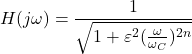Where,
n = order of the filter,
ω = operating frequency (passband frequency) of circuit
ωC = Cut-off frequency
ε = maximum passband gain = Amax

The below equation is used to find the value of ε.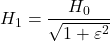Where,
H1 = minimum passband gain
H0 = maximum passband gain

## First-order Lowpass Butterworth Filter

The lowpass filter is a filter that allows the signal with the frequency is lower than the cutoff frequency and attenuates the signals with the frequency is more than cutoff frequency.

In the first-order filter, the number of reactive components is only one. The below figure shows the circuit diagram of the first-order lowpass Butterworth filter.

The low pass Butterworth filter is an active Low pass filter as it consists of the op-amp. This op-amp operates on non-inverting mode. Hence, the gain of the filter will decide by the resistor R1 and RF. And the cutoff frequency decides by R and C.

Now, if you apply the voltage divider rule at point Va and find the voltage across a capacitor. It is given as;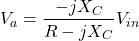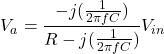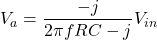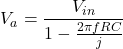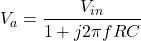Because of the non-inverting configuration of an op-amp,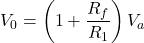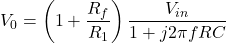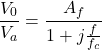Where,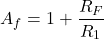Af = Gain of filter in Passband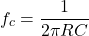fc = Cutoff Frequency
f = Operating Frequency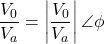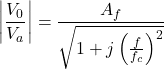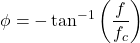1. At very low frequency, f<<fc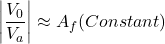2. At cutoff frequency, f= fc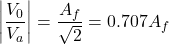3. At high frequency, f> fc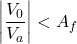The below figure shows the frequency response of first-order lowpass Butterworth filter.

## Second-order Butterworth Filter

The second-order Butterworth filter consists of two reactive components. The circuit diagram of a second-order low pass Butterworth filter is as shown in the below figure.

In this type of filter, resistor R and RF are the negative feedback of op-amp. And the cutoff frequency of the filter decides by R2, R3, C2, and C3.

The second-order lowpass Butterworth filter consists of two back-to-back connected RC networks. And RL is the load resistance.

First-order and second-order Butterworth filters are very important. Because we can get higher-order Butterworth filter by just cascading of the first-order and second-order Butterworth filters.

Let’s analyse the circuit of second-order Butterworth filter,

Apply Kirchhoff’s Current Law at point V1.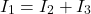(1)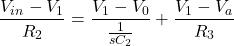Using potential divider rule at point Va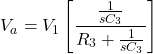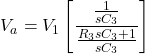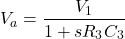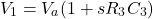Put the value of V1 in equation-(1)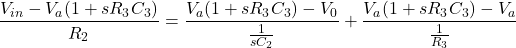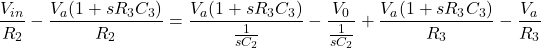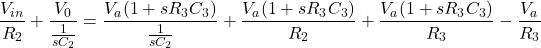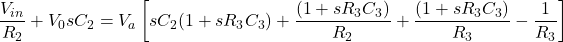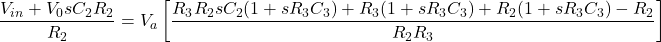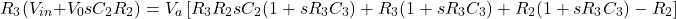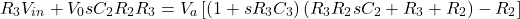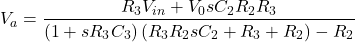Because of the non-inverting configuration of an op-amp,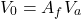Where,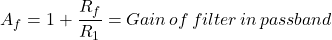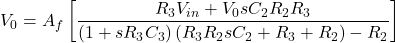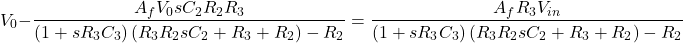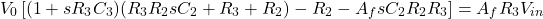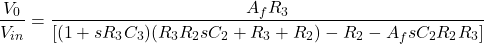Rearrange this equation,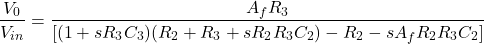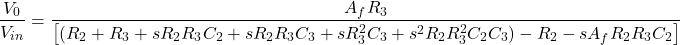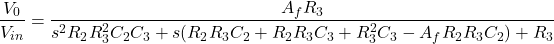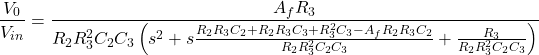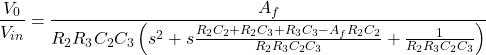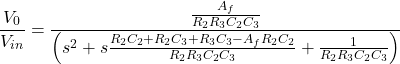Compare this equation with the standard form transfer function for second-order Butterworth filter. And that is,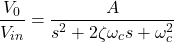By comparing above equations, we can find the equation of cutoff frequency and overall gain for the second-order lowpass Butterworth filter.

The gain of filter is,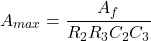And the Cutoff frequency of filter is ,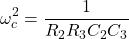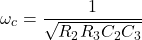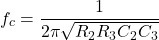Now, if we consider the value of R2 is same as R3 and the value of C2 is same as C3.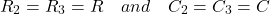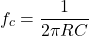Now if we put above values in transfer function,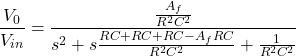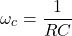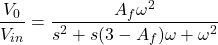From above equation, the quality factor Q is equal to,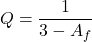We can say that, the quality factor is only depends on the gain of filter. And the value of gain should not more than 3. If the value of gain is more than 3, the system will be unstable.

The value of quality factor is 0.707 for the Butterworth filter. And if we put this value in equation of quality factor, we can find the value of gain.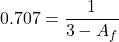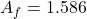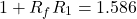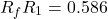While designing the second-order Butterworth filter above relation must be satisfy. The frequency response of this filter is as shown in below figure.

## Third-order Lowpass Butterworth Filter

Third-order lowpass Butterworth filter can design by cascading the first-order and second-order Butterworth filter.

The below figure shows the circuit diagram of the third-order lowpass Butterworth filter.

In this figure, the first part shows the first-order lowpass Butterworth filter, and the second part shows the second-order lowpass Butterworth filter.

But in this condition, the voltage gain of the first part is optional and it can be set at any value. Therefore, the first op-amp is not taking part in voltage gain. Hence, the figure for the third-order low pass filter can be expressed as below figure also;

The voltage gain of a second-order filter affects the flatness of frequency response. If the gain of the second-order filter is kept at 1.586, the gain will down 3db for each part. So, the overall gain will down 6dB at the cutoff frequency.

By increasing the voltage gain of the second-order filter, we can offset the cumulative loss of voltage gain.

In the third-order Butterworth filter, the rate of a roll-off period is -60dB/decade. And the frequency response of this filter is nearer to the ideal Butterworth filter compared to the first and second-order filters. The frequency response of this filter is as shown in the below figure.

## Fourth-order Lowpass Butterworth Filter

Fourth-order Butterworth filter is established by the cascade connection of two second-order low pass Butterworth filters. The circuit diagram of the fourth-order lowpass Butterworth filter is as shown in the below figure.

If the gain of both filters is set at 1.586, the voltage gain will be down 6 dB at the cutoff frequency. We can get a more flat response by choosing different values of voltage gain for both stages. According to the advanced research, we get maximum flat response, if we use the voltage gain 1.152 for the first stage and 2.235 for the second stage.

The below figure shows the frequency response of the fourth-order lowpass Butterworth filter.

## Difference Between Butterworth and Chebyshev Filter

The Chebyshev filter has a steeper roll-off than the Butterworth filter. But it consists of ripples in the passband (type-1) or stopband (type-2). Type-1 Chebyshev filter is commonly used and sometimes it is known as only “Chebyshev filter”. Type-2 filter is also known as “Inverse Chebyshev filter”.

The difference between the Butterworth filter and Chebyshev filter is as shown in the below table.

## Butterworth Filter Applications

The applications of a Butterworth filter are listed below:

• Because of the maximal flat frequency response in the passband, it is used as an anti-aliasing filter in data converter applications.
• The Butterworth filter is used in the audio processing application. An efficient audio noise reduction tool can be developed using a Butterworth filter.
• It is also used in various communication and control systems.
• It is used in radar to design the display of radar target tracking.
• It is used for motion analysis.

Want To Learn Faster? 🎓
Get electrical articles delivered to your inbox every week.
No credit card required—it’s 100% free.